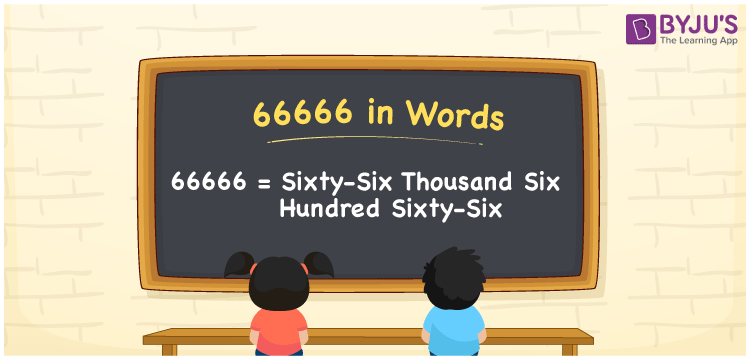# 66666 in words

66666 in words is written as Sixty Six Thousand Six Hundred and Sixty Six. In 66666, the first 6 has a place value of ten thousand, second 6 is in the place value of thousand, third 6 is in the place value of hundred, fourth 6 is in the place value of ten and the last 6 is in the place value of one. The article on Place Value gives more information. The number 66666 is used in expressions that relate to money, distance, social media views, and many more. For example, “In the number Sixty Six Thousand Six Hundred and Sixty Six, the only digit is 6.” Another example “For a festive sale, the smart TV price was reduced to Sixty Six Thousand Six Hundred and Sixty Six rupees.”

 66666 in words Sixty Six Thousand Six Hundred and Sixty Six Sixty Six Thousand Six Hundred and Sixty Six in Numbers 66666

## 66666 in English Words## How to Write 66666 in Words?

We can convert 66666 to words using a place value chart. The number 66666 has 5 digits, so let’s make a chart that shows the place value up to 5 digits.

 Ten thousand Thousands Hundreds Tens Ones 6 6 6 6 6

Thus, we can write the expanded form as:

6 × Ten thousand + 6 × Thousand + 6 × Hundred + 6 × Ten + 6 × One

= 6 × 10000 + 6 × 1000 + 6 × 100 + 6 × 10 + 6 × 1

= 66666.

= Sixty Six Thousand Six Hundred and Sixty Six.

66666 is the natural number that is succeeded by 66665 and preceded by 66667.

66666 in words – Sixty Six Thousand Six Hundred and Sixty Six.

Is 66666 an odd number? – No.

Is 66666 an even number? – Yes.

Is 66666 a perfect square number? – No.

Is 66666 a perfect cube number? – No.

Is 66666 a prime number? – No.

Is 66666 a composite number? – Yes.

## Solved Example

1. Write the number 66666 in expanded form

Solution: 6 x 10000 + 6 x 1000 + 6 x 100 + 6 x 10 + 6 x 1

We can write 66666 = 60000 + 6000 + 600 + 60 + 6

= 6 x 10000 + 6 x 1000 + 6 x 100 + 6 x 10 + 6 x 1.

## Frequently Asked Questions on 66666 in words

Q1

### How to write the number 66666 in words?

66666 in words is written as Sixty Six Thousand Six Hundred and Sixty Six.
Q2

### Is 66666 a prime number?

No. 66666 is not a prime number.
Q3

### Is 66666 divisible by 10?

No. 66666 is not divisible by 10.